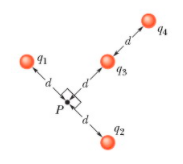# Problem: In the figure the four particles are fixed in place and have charges q1  = q2 = 3e, q3 = 2e and q4 = -9e. Distance d = 2.17 µm. What is the magnitude of net electric field at point P due to the particles?

###### FREE Expert Solution

In this problem, we are dealing with electric fields due to point charges.

Electric field:

$\overline{){\mathbf{E}}{\mathbf{=}}\frac{\mathbf{k}\mathbf{q}}{{\mathbf{r}}^{\mathbf{2}}}}$

80% (175 ratings)###### Problem Details

In the figure the four particles are fixed in place and have charges q1  = q2 = 3e, q3 = 2e and q4 = -9e. Distance d = 2.17 µm. What is the magnitude of net electric field at point P due to the particles?